# Chapter 10 Map Algebra

Dana Tomlin is credited with defining a framework for the analysis of field data stored as gridded values (i.e. rasters). He coined this framework map algebra. Though gridded data can be stored in a vector format, map algebra is usually performed on raster data. Map algebra operations and functions are broken down into four groups: local, focal, zonal and global. Each is explored in the following sections.

## 10.1 Local operations and functions

Local operations and functions are applied to each individual cell and only involve those cells sharing the same location.

For example, if we start off with an original raster, then multiply it by 2 then add 1, we get a new raster whose cell values reflect the series of operations performed on the original raster cells. This is an example of a unary operation where just one single raster is involved in the operation.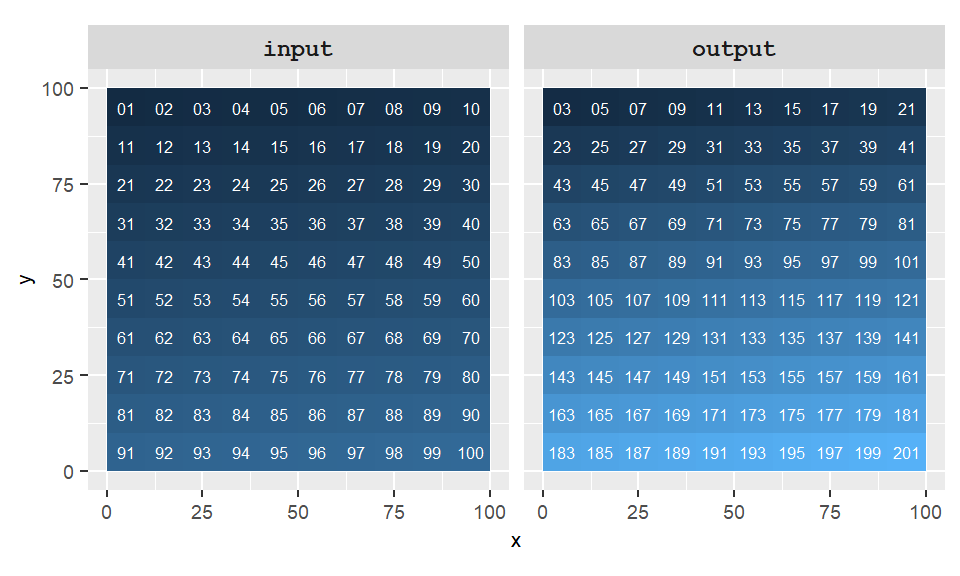Figure 10.1: Example of a local operation where output=(2 * raster + 1).

More than one raster can be involved in a local operation. For example, two rasters can be summed (i.e. each overlapping pixels are summed) to generate a new raster.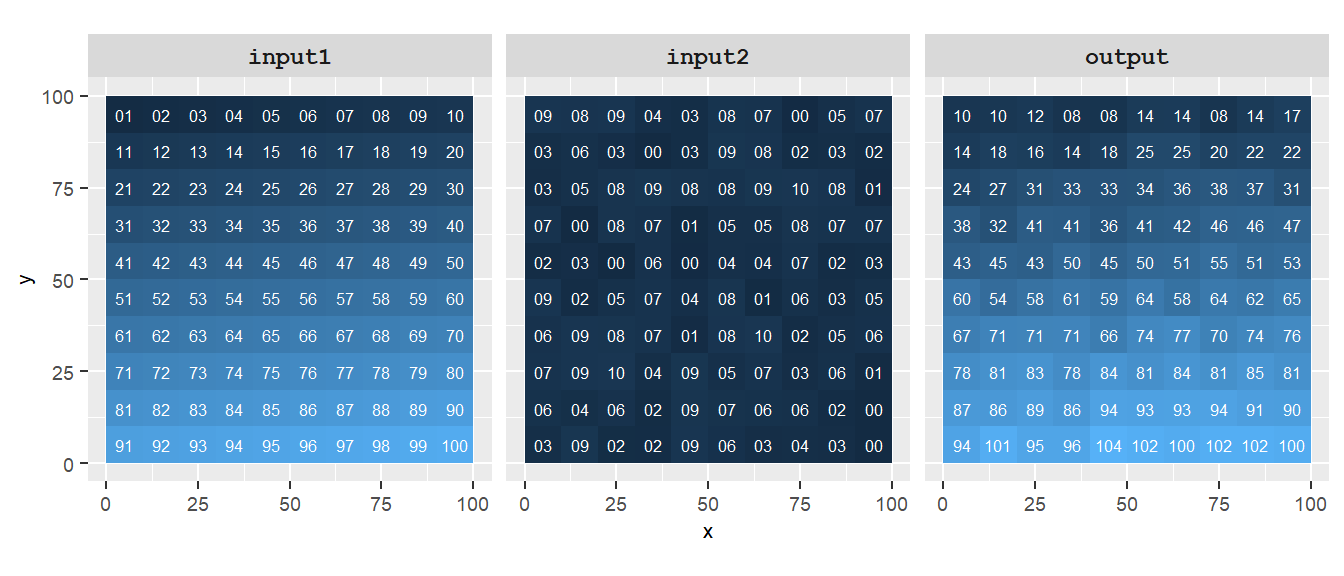Figure 10.2: Example of a local operation where output=(raster1+raster2). Note how each cell output only involves input raster cells that share the same exact location.

Local operations also include reclassification of values. This is where a range of values from the input raster are assigned a new (common) value. For example, we might want to reclassify the input raster values as follows:

Original values Reclassified values
0-25 25
26-50 50
51-75 75
76-100 100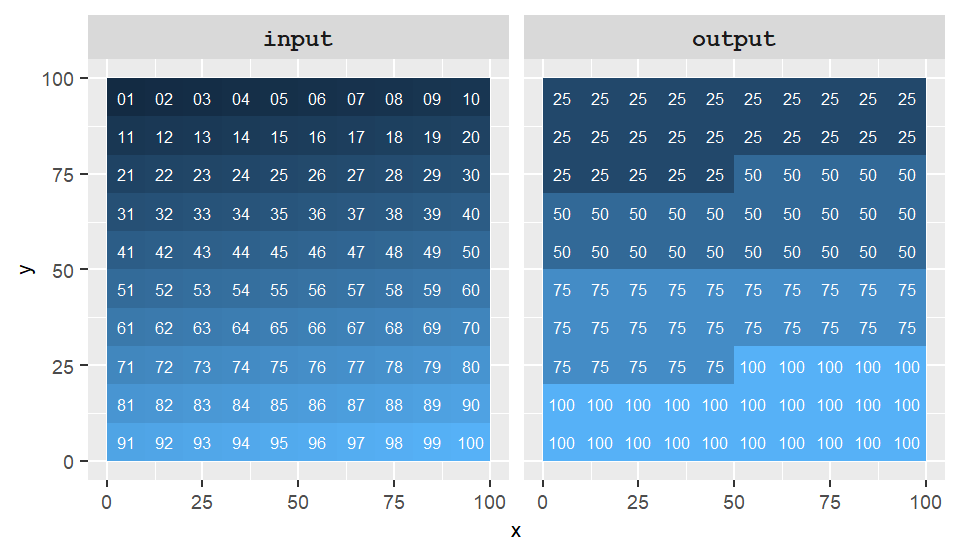Figure 10.3: Example of a local operation where the output results from the reclassification of input values.

## 10.2 Focal operations and functions

Focal operations are also referred to as neighborhood operations.

Focal operations assign to the output cells some summary value (such as the mean) of the neighboring cells from the input raster. For example, a cell output value can be the average of all 9 neighboring input cells (including the center cell); this acts as a smoothing function.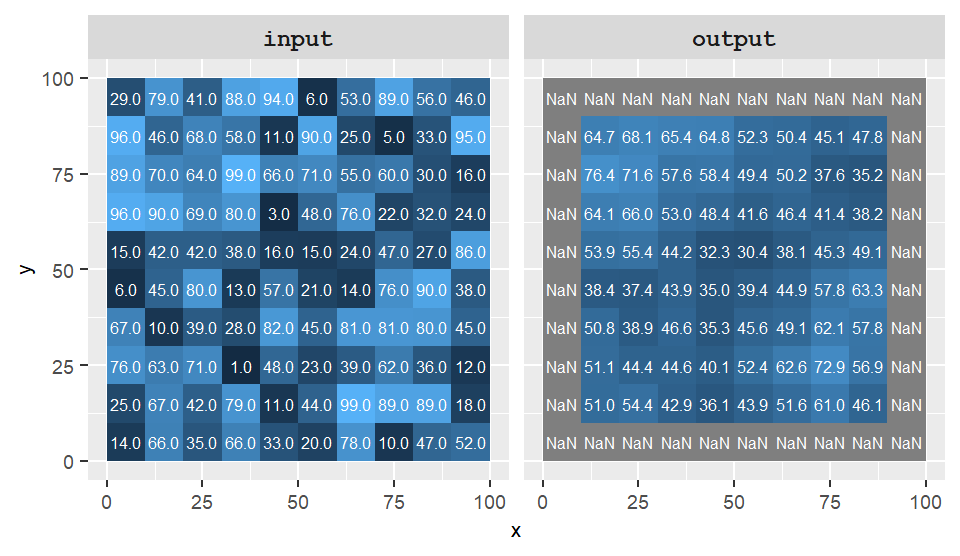Figure 10.4: Example of a focal operation where the output cell values take on the average value of neighboring cells from the input raster. Focal cells surrounded by non-existent cells are assigned an NA in this example.

Notice how, in the above example, the edge cells from the output raster have been assigned a value of NA (No Data). This is because cells outside of the extent have no value. Some GIS applications will ignore the missing surrounding values and just compute the average of the available cells as demonstrated in the next example.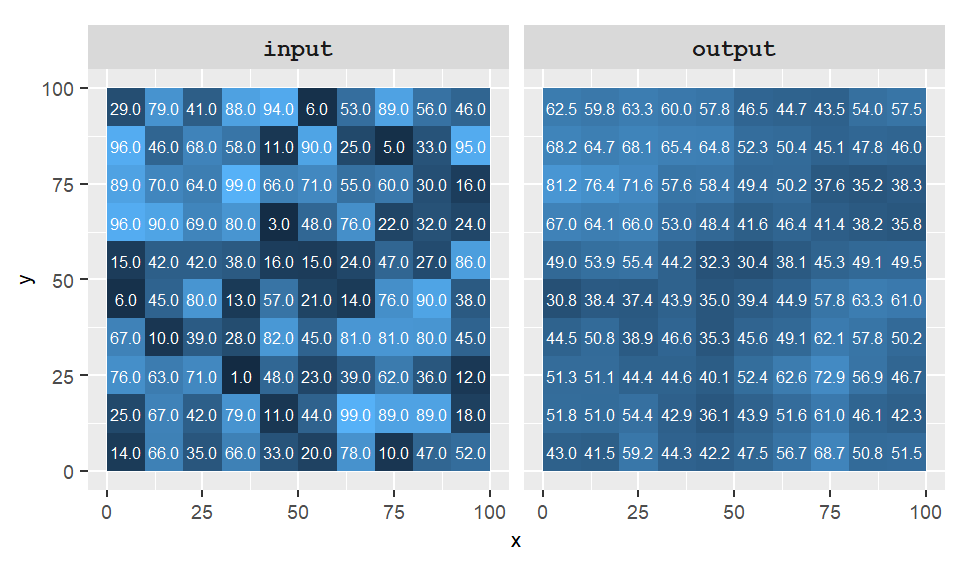Figure 10.5: Example of a focal operation where the output cell values take on the average value of neighboring cells from the input raster. Surrounding non-existent cells are ignored.

Focal (or neighbor) operations require that a window region (a kernel) be defined. In the above examples, a simple 3 by 3 kernel (or window) was used in the focal operations. The kernel can take on different dimensions and shape such as a 3 by 3 square where the central pixel is ignored (thus reducing the number of neighbors to 8) or a circular neighbor defined by a radius.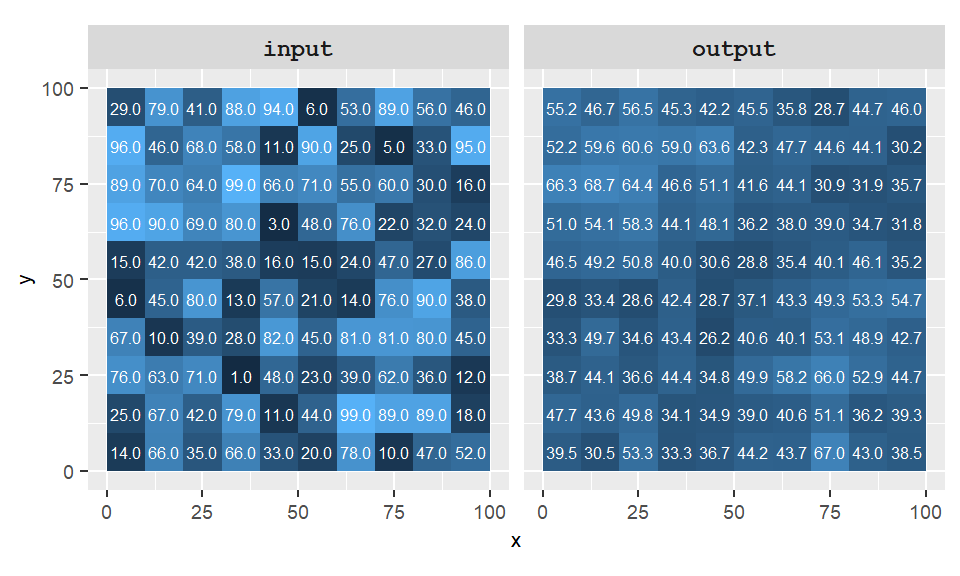Figure 10.6: Example of a focal operation where the kernel is defined by a 3 by 3 cell without the center cell and whose output cell takes on the average value of those neighboring cells.

In addition to defining the neighborhood shape and dimension, a kernel also defines the weight each neighboring cell contributes to the summary statistic. For example, all cells in a 3 by 3 neighbor could each contribute 1/9th of their value to the summarized value (i.e. equal weight). But the weight can take on a more complex form defined by a function; such weights are defined by a kernel function. One popular function is a Gaussian weighted function which assigns greater weight to nearby cells than those further away.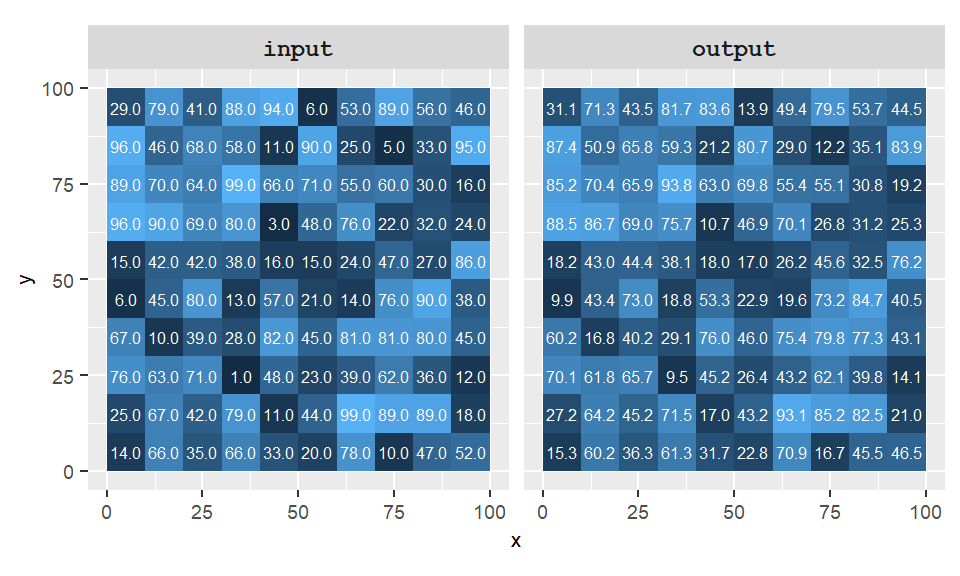Figure 10.7: Example of a focal operation where the kernel is defined by a Gaussian function whereby the closest cells are assigned a greater weight.

## 10.3 Zonal operations and functions

A zonal operation computes a new summary value (such as the mean) from cells aggregated for some zonal unit. In the following example, the cell values from the raster layer are aggregated into three zones whose boundaries are delineated in red. Each output zone shows the average value of the cells within that zone.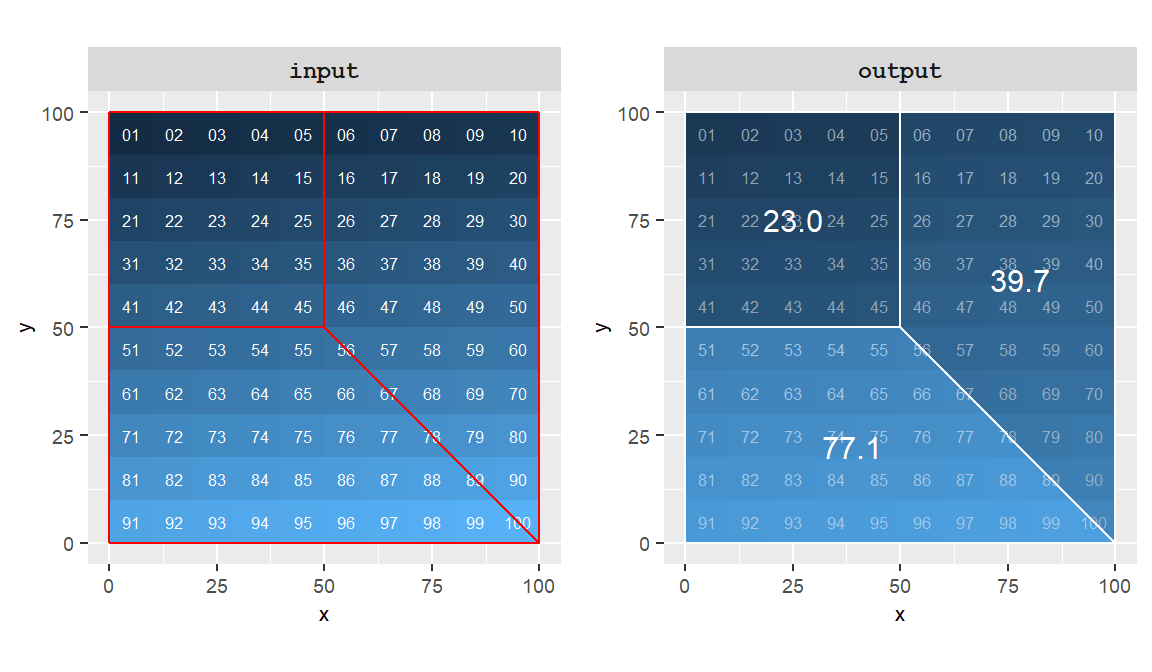Figure 10.8: Example of a zonal operation where the cell values are averaged for each of the three zones delineated in red.

This technique is often used with rasters derived from remotely sensed imagery (e.g. NDVI) where areal units (such as counties or states) are used to compute the average cell values from the raster.

## 10.4 Global operations and functions

Global operations and functions may make use of some or all input cells when computing an output cell value. An example of a global function is the Euclidean Distance tool which computes the shortest distance between a pixel and a source (or destination) location. In the following example, a new raster assigns to each cell a distance value to the closest cell having a value of 1 (there are just two such cells in the input raster).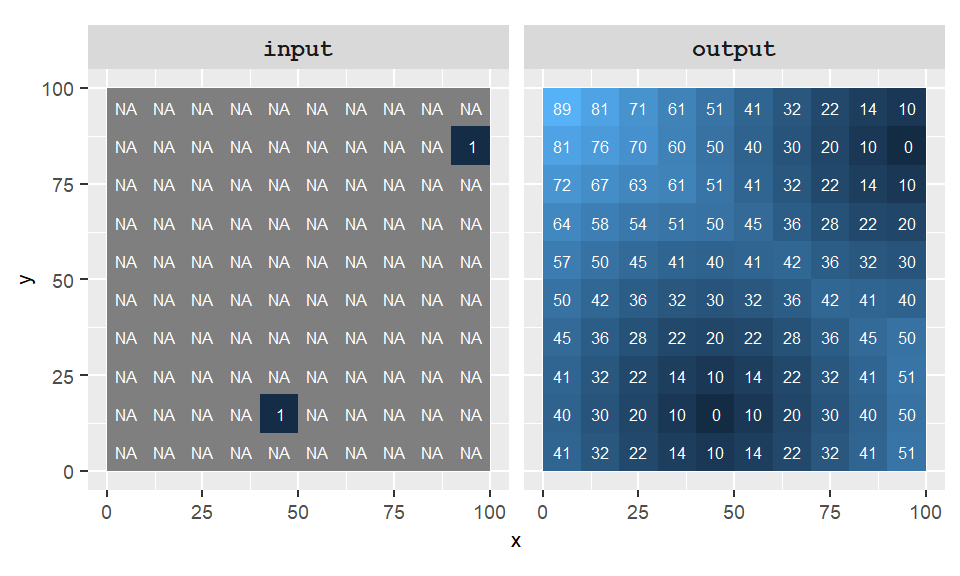Figure 10.9: Example of a global function: the Euclidean distance. Each pixel is assigned its closest distance to one of the two source locations (defined in the input layer).

Global operations and functions can also generate single value outputs such as the overall pixel mean or standard deviation.

Another popular use of global functions is in the mapping of least-cost paths where a cost surface raster is used to identify the shortest path between two locations which minimizes cost (in time or money).

## 10.5 Operators and functions

Operations and functions applied to gridded data can be broken down into three groups: mathematical, logical comparison and Boolean.

### 10.5.1 Mathematical operators and functions

Two mathematical operators have already been demonstrated in earlier sections: the multiplier and the addition operators. Other operators include division and the modulo (aka the modulus) which is the remainder of a division. Mathematical functions can also be applied to gridded data manipulation. Examples are square root and sine functions. The following table showcases a few examples with ArcGIS and R syntax.

Operation ArcGIS Syntax R Syntax Example
Addition + + R1 + R2
Subtraction - - R1 - R2
Division / / R1 / R2
Modulo Mod() %% Mod(R1, 100), R1 %% 10
Square root SquareRoot() sqrt() SquareRoot(R1), sqrt(R1)

### 10.5.2 Logical comparison

The logical comparison operators evaluate a condition then output a value of 1 if the condition is true and 0 if the condition is false. Logical comparison operators consist of greater than, less than, equal and not equal.

Logical comparison Syntax
Greater than >
Less than <
Equal ==
Not equal !=

For example, the following figure shows the output of the comparison between two rasters where we are assessing if cells in R1 are greater than those in R2 (on a cell-by-cell basis).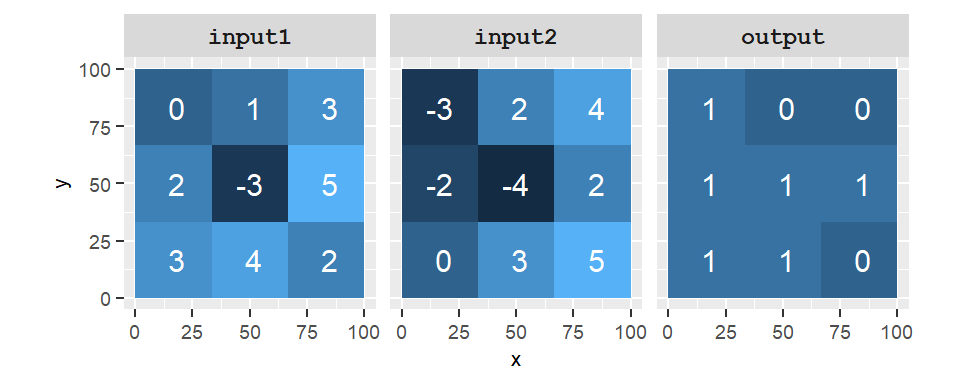Figure 10.10: Output of the operation R1 > R2. A value of 1 in the output raster indicates that the condition is true and a value of 0 indicates that the condition is false.

When assessing whether two cells are equal, some programming environments such as R and ArcMap’s Raster Calculator require the use of the double equality syntax, ==, as in R1 == R2. In these programming environments, the single equality syntax is usually interpreted as an assignment operator so R1 = R2 would instruct the computer to assign the cell values in R2 to R1 (which is not what we want to do here).

Some applications make use of special functions to test a condition. For example, ArcMap has a function called Con(condition, out1, out2) which assigns the value out1 if the condition is met and a value of out2 if it’s not. For example, ArcMap’s raster calculator expression

Con( R1 > R2, 1, 0)

outputs a value of 1 if R1 is greater than R2 and 0 if not. It generates the same output as the one shown in the above figure. Note that in most programming environments (including ArcMap), the expression R1 > R2 produces the same output because the value 1 is the numeric representation of TRUE and 0 that of FALSE.

### 10.5.3 Boolean (or Logical) operators

In map algebra, Boolean operators are used to compare conditional states of a cell (i.e. TRUE or FALSE). The three Boolean operators are AND, OR and NOT.

Boolean ArcGIS R Example
AND & & R1 & R2
OR | | R1 | R2
NOT ~ ! ~R2, !R2

A “TRUE” state is usually encoded as a 1 or any non-zero integer while a “FALSE” state is usually encoded as a 0.

For example, if cell1=0 and cell2=1, the Boolean operation cell1 AND cell2 results in a FALSE (or 0) output cell value. This Boolean operation can be translated into plain English as “are the cells 1 and 2 both TRUE?” to which we answer “No they are not” (cell1 is FALSE). The OR operator can be interpreted as “is x or y TRUE?” so that cell1 OR cell2 would return TRUE. The NOT interpreter can be interpreted as “is x not TRUE?” so that NOT cell1 would return TRUE.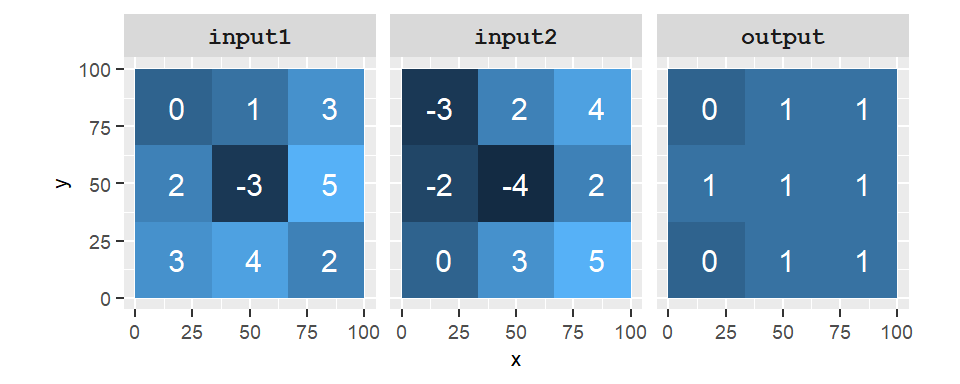Figure 10.11: Output of the operation R1 AND R2. A value of 1 in the output raster indicates that the condition is true and a value of 0 indicates that the condition is false. Note that many programming environments treat any none 0 values as TRUE so that -3 AND -4 will return TRUE.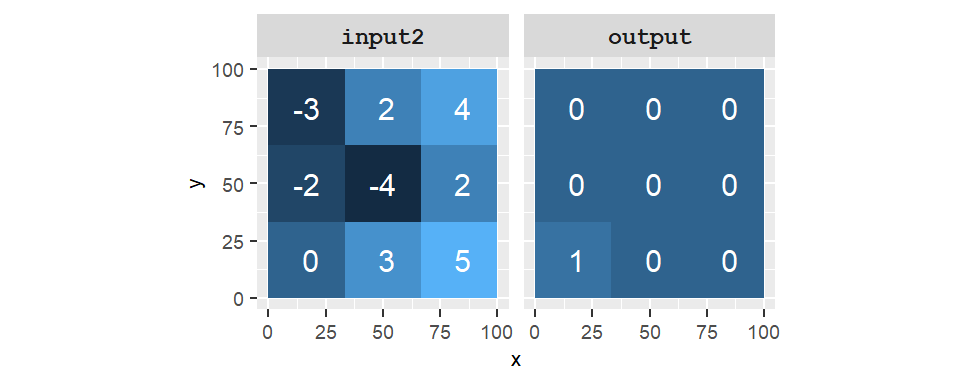Figure 10.12: Output of the operation NOT R2. A value of 1 in the output raster indicates that the input cell is NOT TRUE (i.e. has a value of 0).

### 10.5.4 Combining operations

Both comparison and Boolean operations can be combined into a single expression. For example, we may wish to find locations (cells) that satisfy requirements from two different raster layers: e.g. 0<R1<4 AND R2>0. To satisfy the first requirement, we can write out the expression as (R1>0) & (R1<4). Both comparisons (delimited by parentheses) return a 0 (FALSE) or a 1 (TRUE). The ampersand, &, is a Boolean operator that checks that both conditions are met and returns a 1 if yes or a 0 if not. This expression is then combined with another comparison using another ampersand operator that assesses the criterion R2>0. The amalgamated expression is thus ((R1>0) & (R1<4)) & (R2>0).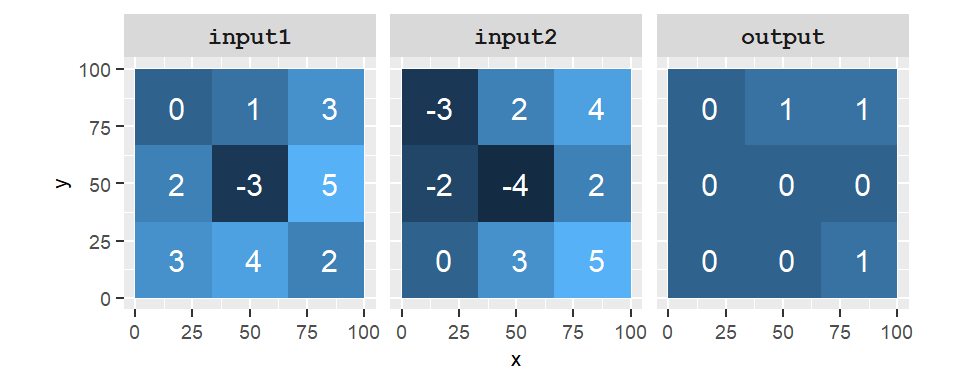Figure 10.13: Output of the operation ((R1>0) & (R1<4)) & (R2>0). A value of 1 in the output raster indicates that the condition is true and a value of 0 indicates that the condition is false.

Note that most software environments assign the AND Boolean operator the ampersand character, &.

### References

Tomlin, Dana C. 1990. GIS and Cartographic Modeling. New Jersey: Prentice Hall.